# If the ratio of boys to girls is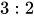and there are 25 students in a class, how do you make equal ratios to show how many students in the class are boys and how many are girls?Rupinder

Solution:

Concept:When any two or more quantities are given in the form of ratio then the actual distribution value is calculated as follows:

Let us suppose the quantity A and B in a mixture of x quantity is in the ratio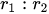then the amount of A is obtained equal to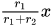and the amount of B is obtained equal to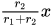.

Calculation:

Step:1 The ratio of boys to girls is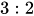and the total number of students is 25.

Step:2 Then by the concept, the number of boys in the class is calculated as follows:Step:3 Similarly, the number of girls in the class is calculated as follows:Hence, the number of boys are 15 and number of girls are 10, in a class of 25 students.

Answer:There are 15 boys and 10 girls in a class of 25 students.

Similar Problems:

Problem 1:If 15 toffees are distributive to two children in the ratio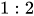then how many toffees does each one get?

Solution:

Step:1 The ratio of toffees distributed to two children isand the total number of toffees is 25.

Step:2 Then by the concept, the number of toffeesfirst child receives is calculated as follows:Step:3 Similarly, the number of toffees second child receives is calculated as follows:Hence, the first one gets 5 toffees and the second gets 10 toffees.

Answer:The first one gets 5 toffees and the second gets 10 toffees.

Problem 2:If 12 litres of milk water mixturecontains milk and water in the ratio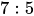then how much amount of milk is present in the mixture?

Solution:

Step:1 The ratio of milk and water in the mixture of 12 litresis.

Step:2 Then by the concept, the amount of milk is calculated as follows:Hence, the amount of milk present in the mixture is 7 litres.

Answer: The mixture contains 7 litres of milk.

### Need homework help now?

tutors online

Related Questions
##### (t) in °C of the pot of soup at time t (in The initial temperature of a pot of soup is 80°C. The temperature minutes) satisfies the differential equation dT di T-20 10 (a) Solve the differential equation, and find an explicit expression for the function (). By separating variables, integrating and manipulating arbitrary constants, . We are also told that so that and so . ...
Notes
Me

OR

Don't have an account?

Join OneClass

Access over 10 million pages of study
documents for 1.3 million courses.

Join to view

OR

By registering, I agree to the Terms and Privacy Policies
Just a few more details

So we can recommend you notes for your school.